# AP Board 6th Class Maths Solutions Chapter 6 Basic Arithmetic Ex 6.4

AP State Syllabus AP Board 6th Class Maths Solutions Chapter 6 Basic Arithmetic Ex 6.4 Textbook Questions and Answers.

## AP State Syllabus 6th Class Maths Solutions 6th Lesson Basic Arithmetic Ex 6.4

Question 1.
Express each of the following percents as fractions in the simplest form,
a) 15%
b) 35%
c) 50%
d) 75%
a) Given percent is 15%
= $$\frac{15}{100}$$ = $$\frac{3}{20}$$ (fraction form) [∵ To express a percentage as a fraction first drop the symbol % and then divide it by 100.]

b) Given percent is 35%
= $$\frac{35}{100}$$ = $$\frac{7}{20}$$ (fraction form)

c) Given percent is 50%
= $$\frac{50}{100}$$ = $$\frac{1}{2}$$ (fraction form)

d) Given percent is 75%
= $$\frac{75}{100}$$ = $$\frac{3}{4}$$ (fraction form)Question 2.
Express each of the following fractions as percents.
a) $$\frac{15}{2}$$
b) 8$$\frac{1}{4}$$
c) 5$$\frac{3}{4}$$
d) 3$$\frac{1}{3}$$
a) Given number $$\frac{15}{2}$$ is in fraction form.
To convert the fraction into percent we have to multiply the fraction by 100%
$$\frac{15}{2}$$ = $$\frac{15}{2}$$ × 100 = 15 × 50 = 750% (percent form)

b) Given number 8$$\frac{1}{4}$$ is in fraction form.
To convert the fraction into percent we have to multiply the fraction by 100%
8$$\frac{1}{4}$$ = $$\frac{33}{4}$$ = $$\frac{33}{4}$$ × 100 = 33 × 25 = 825%,(percent form).

c) Given number 5$$\frac{3}{4}$$ is in fraction form.
To convert the fraction into percent we have to multiply the fraction by 100 and assign the percentage symbol %
5$$\frac{3}{4}$$ = $$\frac{23}{4}$$ × 100 = 23 × 25 = 575% (percent form)

d) Given number is 3$$\frac{1}{3}$$ in fraction form.
To convert the fraction into percent we have to multiply the fraction by 100%
3$$\frac{1}{3}$$ = $$\frac{10}{3}$$ × 100 = $$\frac{1000}{3}$$ = 333$$\frac{1}{3}$$ % (percent form)

Question 3.
Express each of the following ratios as percents.
(a) 3 : 5 (b) 5 : 8 (c) 2.5 : 55 (d) 4 : 36
a) 3 : 5
Given ratio is 3 : 5.
3 : 5 = $$\frac{3}{5}$$ (fraction form)
To convert the fraction into percent we have to multiply the fraction by 100%
$$\frac{3}{5}$$ = $$\frac{3}{5}$$ × 100 = 60% (percent form)b) 5 : 8
Given ratio is 5 : 8
5 : 8 = $$\frac{5}{8}$$ (fraction form)
To convert the fraction into percent we have to multiply the fraction by 100%
$$\frac{3}{5}$$ = $$\frac{3}{5}$$ × 100 = 62 $$\frac{1}{2}$$ % (percent form)

c) 2.5 : 55
Given ratio is 2.5 : 55
2.5 : 55 = $$\frac{2.5}{55}$$
To convert the fraction into percent we have to multiply the fraction by 100%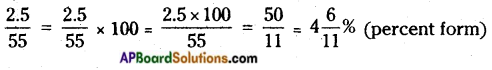d) 4 : 3 6
Given ratio is 4 : 36
4 : 36 = $$\frac{4}{36}$$ (fraction form)
To convert the fraction into percent we have to multiply the fraction by 100%
$$\frac{4}{36}$$ = $$\frac{4}{36}$$ × 100 = $$\frac{100}{9}$$ = 11 $$\frac{1}{9}$$ % (percent form)

Question 4.
Express each of the following percents as ratios in the simplest form.
(a) 12% (b) 25% (c) 45% (d) 84%
a) Given percent is 12%
= $$\frac{12}{100}$$ = $$\frac{3}{25}$$ (fraction form)
= 3 : 25 (ratio form)

b) Given percent is 25%
= $$\frac{25}{100}$$ = $$\frac{1}{4}$$ (fraction form)
= 1 : 4 (ratio form)

c) Given percent is 45%
= $$\frac{45}{100}$$ = $$\frac{9}{20}$$ (fraction form)
= 9 : 20 (ratio form)

d) Given percent is 84%
= $$\frac{84}{100}$$ = $$\frac{21}{25}$$ (fraction form)
= 21 : 25 (ratio form)Question 5.
Express each of the following percents as decimals,
(a) 1% (b) 6% (c) 19% (d) 67%
a) Given percent is 1%
= 1% = $$\frac{1}{100}$$ (fraction form)
= 0.01 (decimal form)

b) Given percent is 6%
= 6% = $$\frac{6}{100}$$ = $$\frac{3}{50}$$ (fraction form)
= 0.06 (decimal form)

c) Given percent is 19%
= 19% = $$\frac{19}{100}$$ (fraction form)
= 0.19 (decimal form)

d) Given percent is 67%
= 67% = $$\frac{67}{100}$$
= 0.67 (decimal form)

Question 6.
Express each of the following decimals as percent.
(a) 0.04 (b) 0.52 (c) 0.125 (d) 0.0006
a) Given decimal number is 0.04.
Convert this into fraction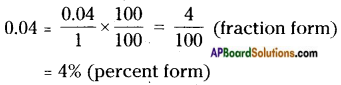b) Given decimal number is 0.52.
Convert this into fraction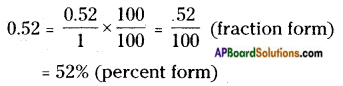c) Given decimal number is 0.125.
Convert this into fraction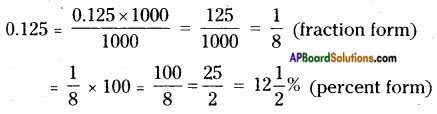d) Given decimal number is 0.0006.
Convert this into fraction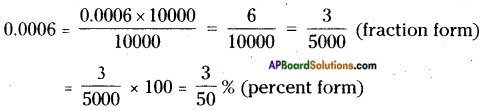Question 7.
Find the number, which is 12$$\frac{1}{2}$$% of 75.
Given 12$$\frac{1}{2}$$% of 75
We know that x% of y = $$\frac{x}{100}$$ × y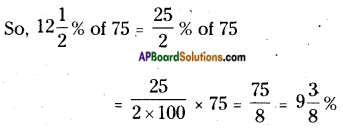(OR)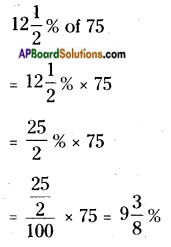Question 8.
Pavani secured 85% marks in mathematics paper. If maximum marks in the paper are 80, find the marks secured by her in that paper.
Given maximum marks of the paper = 80
Percentage of marks secured by Pavani = 85%
Marks secured by Pavani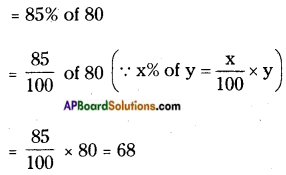∴ Marks secured by Pavani = 68Question 9.
Siva spends 78% of his monthly income. If he saves Rs. 7,700/- per month, what is his monthly income?
⇒ $$\frac{1}{2}$$ × x = 7700
⇒ x = $$\frac{7700×100}{2}$$